The SURVEYLOGISTIC Procedure

### Model Options

Model options can be specified after a slash (/). Table 85.6 summarizes the options available in the MODEL statement.

Table 85.6 MODEL Statement Options

Option

Description

Model Specification Options

Suppresses intercept(s)

Specifies offset variable

Convergence Criterion Options

Specifies absolute function convergence criterion

Specifies relative function convergence criterion

Specifies relative parameter convergence criterion

Specifies maximum number of iterations

Suppresses checking for infinite parameters

Specifies technique used to improve the log-likelihood function when its value is worse than that of the previous step

Specifies tolerance for testing singularity

Specifies iterative algorithm for maximization

Options for Adjustment to Variance Estimation

Options for Confidence Intervals

Specifiesfor the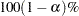confidence intervals

Computes confidence intervals for parameters

Computes confidence intervals for odds ratios

Options for Display of Details

Displays correlation matrix

Displays covariance matrix

Displays exponentiated values of estimates

Displays iteration history

Suppresses "Class Level Information" table

Displays parameter labels

Displays generalizedDisplays standardized estimates

The following list describes these options:

ABSFCONV=value

specifies the absolute function convergence criterion. Convergence requires a small change in the log-likelihood function in subsequent iterations: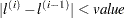where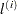is the value of the log-likelihood function at iteration. See the section Convergence Criteria.

ALPHA=value

sets the level of significancefor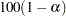% confidence intervals for regression parameters or odds ratios. The valuemust be between 0 and 1. By default,is equal to the value of the ALPHA= option in the PROC SURVEYLOGISTIC  statement, or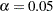if the ALPHA= option is not specified. This option has no effect unless confidence limits for the parameters or odds ratios are requested.

CLODDS

requests confidence intervals for the odds ratios. Computation of these confidence intervals is based on individual Wald tests. The confidence coefficient can be specified with the ALPHA= option.

CLPARM

requests confidence intervals for the parameters. Computation of these confidence intervals is based on the individual Wald tests. The confidence coefficient can be specified with the ALPHA= option.

CORRB

displays the correlation matrix of the parameter estimates.

COVB

displays the covariance matrix of the parameter estimates.

EXPB
EXPEST

displays the exponentiated values (e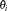) of the parameter estimates
in the "Analysis of Maximum Likelihood Estimates" table for the logit model. These exponentiated values are the estimated odds ratios for the parameters corresponding to the continuous explanatory variables.

FCONV=value

specifies the relative function convergence criterion. Convergence requires a small relative change in the log-likelihood function in subsequent iterations: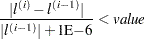whereis the value of the log likelihood at iteration. See the section Convergence Criteria for details.

GCONV=value

specifies the relative gradient convergence criterion. Convergence requires that the normalized prediction function reduction is small: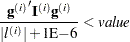whereis the value of the log-likelihood function,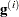is the gradient vector, and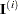the (expected) information matrix. All of these functions are evaluated at iteration. This is the default convergence criterion, and the default value is 1E8. See the section Convergence Criteria for details.

ITPRINT

displays the iteration history of the maximum-likelihood model fitting. The ITPRINT option also displays the last evaluation of the gradient vector and the final change in the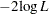.

L=keyword

specifies the link function that links the response probabilities to the linear predictors. You can specify one of the following keywords. The default is LINK=LOGIT.

CLOGLOG

specifies the complementary log-log function. PROC SURVEYLOGISTIC  fits the binary complementary log-log model for binary response and fits the cumulative complementary log-log model when there are more than two response categories. Aliases: CCLOGLOG, CCLL, CUMCLOGLOG.

GLOGIT

specifies the generalized logit function. PROC SURVEYLOGISTIC  fits the generalized logit model where each nonreference category is contrasted with the reference category. You can use the response variable option REF= to specify the reference category.

LOGIT

specifies the cumulative logit function. PROC SURVEYLOGISTIC  fits the binary logit model when there are two response categories and fits the cumulative logit model when there are more than two response categories. Aliases: CLOGIT, CUMLOGIT.

PROBIT

specifies the inverse standard normal distribution function. PROC SURVEYLOGISTIC  fits the binary probit model when there are two response categories and fits the cumulative probit model when there are more than two response categories. Aliases: NORMIT, CPROBIT, CUMPROBIT.

See the section Link Functions and the Corresponding Distributions for details.

MAXITER=n

specifies the maximum number of iterations to perform. By default, MAXITER=25. If convergence is not attained initerations, the displayed output created by the procedure contains results that are based on the last maximum likelihood iteration.

NOCHECK

disables the checking process to determine whether maximum likelihood estimates of the regression parameters exist. If you are sure that the estimates are finite, this option can reduce the execution time when the estimation takes more than eight iterations. For more information, see the section Existence of Maximum Likelihood Estimates.

NODUMMYPRINT

suppresses the "Class Level Information" table, which shows how the design matrix columns for the CLASS variables are coded.

NOINT

suppresses the intercept for the binary response model or the first intercept for the ordinal response model.

OFFSET=name

names the offset variable. The regression coefficient for this variable is fixed at 1.

PARMLABEL

displays the labels of the parameters in the "Analysis of Maximum Likelihood Estimates" table.

RIDGING=ABSOLUTE | RELATIVE | NONE

specifies the technique used to improve the log-likelihood function when its value in the current iteration is less than that in the previous iteration. If you specify the RIDGING=ABSOLUTE option, the diagonal elements of the negative (expected) Hessian are inflated by adding the ridge value. If you specify the RIDGING=RELATIVE option, the diagonal elements are inflated by a factor of 1 plus the ridge value. If you specify the RIDGING=NONE option, the crude line search method of taking half a step is used instead of ridging. By default, RIDGING=RELATIVE.

RSQUARE

requests a generalizedmeasure for the fitted model.

SINGULAR=value

specifies the tolerance for testing the singularity of the Hessian matrix (Newton-Raphson algorithm) or the expected value of the Hessian matrix (Fisher scoring algorithm). The Hessian matrix is the matrix of second partial derivatives of the log likelihood. The test requires that a pivot for sweeping this matrix be at least this value times a norm of the matrix. Values of the SINGULAR= option must be numeric. By default, SINGULAR=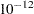.

STB

displays the standardized estimates for the parameters for the continuous explanatory variables in the "Analysis of Maximum Likelihood Estimates" table. The standardized estimate of
is given by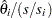, where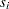is the total sample standard deviation for theth explanatory variable and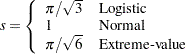For the intercept parameters and parameters associated with a CLASS variable, the standardized estimates are set to missing.

TECHNIQUE=FISHER | NEWTON
TECH=FISHER | NEWTON

specifies the optimization technique for estimating the regression parameters. NEWTON (or NR) is the Newton-Raphson algorithm and FISHER (or FS) is the Fisher scoring algorithm. Both techniques yield the same estimates, but the estimated covariance matrices are slightly different except for the case where the LOGIT link is specified for binary response data. The default is TECHNIQUE=FISHER. If the LINK=GLOGIT option is specified, then Newton-Raphson is the default and only available method. See the section Iterative Algorithms for Model Fitting for details.

specifies an adjustment to the variance estimation for the regression coefficients.

If you do not want to use any variance adjustment, you can specify the VADJUST=NONE option. You can specify the VADJUST=MOREL option for the variance adjustment proposed by Morel (1989).

You can specify the following Morel-options within parentheses after the VADJUST=MOREL option:

ADJBOUND=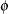sets the upper bound coefficientin the variance adjustment. This upper bound must be positive. By default, the procedure uses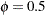. See the section Adjustments to the Variance Estimation for more details on how this upper bound is used in the variance estimation.

DEFFBOUND=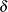sets the lower bound of the estimated design effect in the variance adjustment. This lower bound must be positive. By default, the procedure uses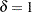. See the section Adjustments to the Variance Estimation for more details about how this lower bound is used in the variance estimation.

XCONV=value

specifies the relative parameter convergence criterion. Convergence requires a small relative parameter change in subsequent iterations: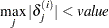where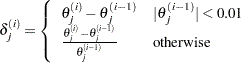and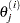is the estimate of theth parameter at iteration. See the section Iterative Algorithms for Model Fitting.Previous Page | Next Page | Top of Page